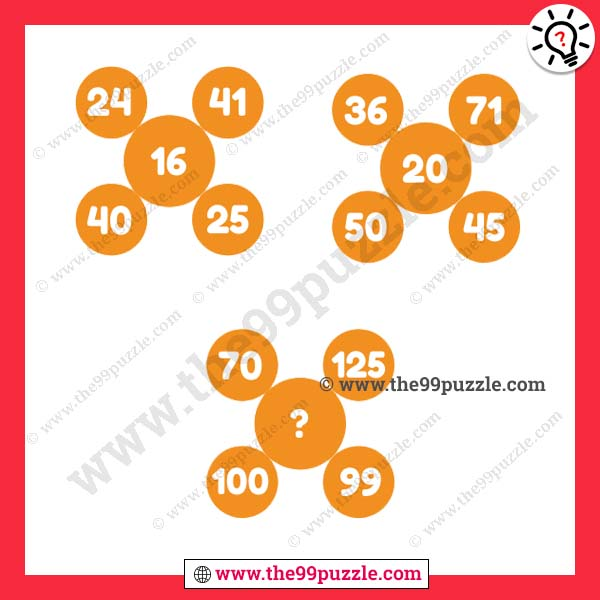# Logic puzzles with numbers for school students – Puzz267

These types of puzzles are fun and tricky. Test your math skills to solve this brainteaser puzzle. You learn maths shortcut tricks and math logic from it. Sometimes this math puzzle gets very hard but when you crack the math logic this is very easy to solve. If you understand the logic you can easily find out the value of the logical thinking viral math equations. In this math equation puzzle, some equations give here. All equations are in the same math logic. You have to crack the logic and replace the question mark. Mathematical logic puzzles with numbers for school students are asked by a math teacher who wants to test their student’s logic skills.###### Explanation:

1st Problem (Top Left) = (40-24)+(41-25) = 16+16 = 32÷2 = 16

2nd Problem (Top Right) = (50-36)+(71-45) = 14+26 = 40÷2 = 20

3rd Problem (Bottom) = (100-70)+(125-99) = 30+26 = 56÷2 = 28